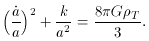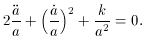D. Matter Dominated Models

The present epoch is best described by a matter dominated universe, so it is perhaps best to explore this model first. Again, matter domination corresponds to a non-relativistic, homogeneous, isotropic `dust' filled universe with zero pressure. By setting p = 0 in (15) and incorporating theterm into a total density,T =+/ (8G), the Friedmann equations for a matter dominated universe emerge,(28)(29)

Again, the value of k describes the geometry of the space. Equation (29) is actually obtained by combining (14) and (15) and is often called the acceleration equation.

The idea of the total density,T, might be a bit confusing since it has been stated that the model is matter dominated. Although this is true, there can still be a small contribution in the form of radiation and other forms of energy, such as dark matter. The point is that any of these should be much less thanM for the model to be accurate. It will also be seen thatM can also be broken into different contributions as tacitly stated in the previous remark about dark matter. For the remainder of this section we taketo meanT to keep the notation as simple as possible.